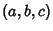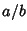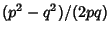## Pythagorean Fraction

Given a Pythagorean Triple, the fractionsandare called Pythagorean fractions. Diophantus showed that the Pythagorean fractions consist precisely of fractions of the form.

References

Conway, J. H. and Guy, R. K. ``Pythagorean Fractions.'' In The Book of Numbers. New York: Springer-Verlag, pp. 171-173, 1996.